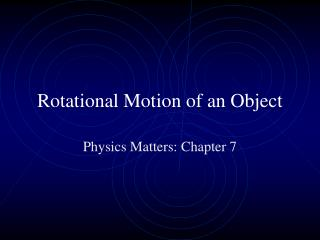DownloadDownload PresentationRotational Motion of an Object

# Rotational Motion of an Object

Télécharger la présentation## Rotational Motion of an Object

- - - - - - - - - - - - - - - - - - - - - - - - - - - E N D - - - - - - - - - - - - - - - - - - - - - - - - - - -
##### Presentation Transcript

1. Rotational Motion of an Object Physics Matters: Chapter 7

2. Rotational Motion • All Spinning Objects • Axis of Rotation • The line about which everything rotates. • Speed of Rotation • Period of rotation • The time of a single complete rotation (T) • Frequency of rotation • The number of cycles completed in a given time (f = hertz) • Period = 1/Frequency or Frequency = 1/Period • T= 1/f f=1/T

3. Spinning • Angular speed •  = s/r ( is measured in radians) • For 360 degreed, s = 2r • 3600 = 2 radians • Angular speed = 2f

4. Torque • Tangential force being applied times the perpendicular distance from the axis of rotation •  = Ftr

5. Angular Momentum • Moment of inertia • In general, the farther away a mass is from the axis the greater its moment of inertia is. • I = kmr2

6. Angular Momentum (Cont.) • Momentum of inertia times angular speed • L = I  • Conservation of Angular momentum • Direction of Rotation • The right hand rule

7. Center of GravityCenter of Mass "All of science is nothing more than the refinement of everyday thinking."-- Albert Einstein

8. Center of Gravity • Point of an object located at the average position of weight.

9. Center of Mass • The Average position of matter

10. Toppling • Toppling occurs when the center of gravity extends beyond the support base.

11. Stability • Unstable – CG is lowered with displacement • Stable – work must be done to raise the CG • Neutral – displacement neither raises or lowers the CG

12. Objects rotate around their center of gravity. Let’s try some activities!!